Resources

# Using the Thermal modeling of power switches

This tutorial explores the "Thermal modeling of power switches" feature of SIMBA.

• carry out thermal modelling structure of a system
• calculate conduction and switching losses
• calculate components temperature
• estimate efficiency of power converter
• design cooling system such as heatsink

## How to use "Thermal modeling of power switches" capability for any circuits

To describe this feature, the example of "DC-DC Buck-Chopper (Thermal)" circuit is considered. This schematic allows to:

• set up thermal modelling techniques
• calculates both conduction and switching losses of one IGBT
• determine junction temperature of the semi conductor
• implement thermal network for the heatsink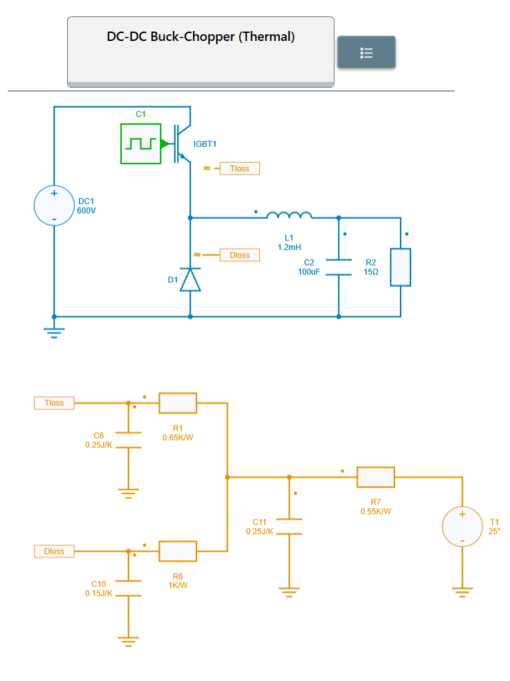Step 1: Firstly, let's observe the "thermal" library: there are 3 important items:

• sources
• RC
• probes

The "semiconductors" library is also really important because it gather the major components such as "diode", "IGBT", and "MOSFET" allowing to set up thermal datas. Those 2 combined libraries allows the user to implement its own thermal circuit for calculating losses, temperature, and heat flow. Also a heatsink could be simulated by using resistor and capacitor networks.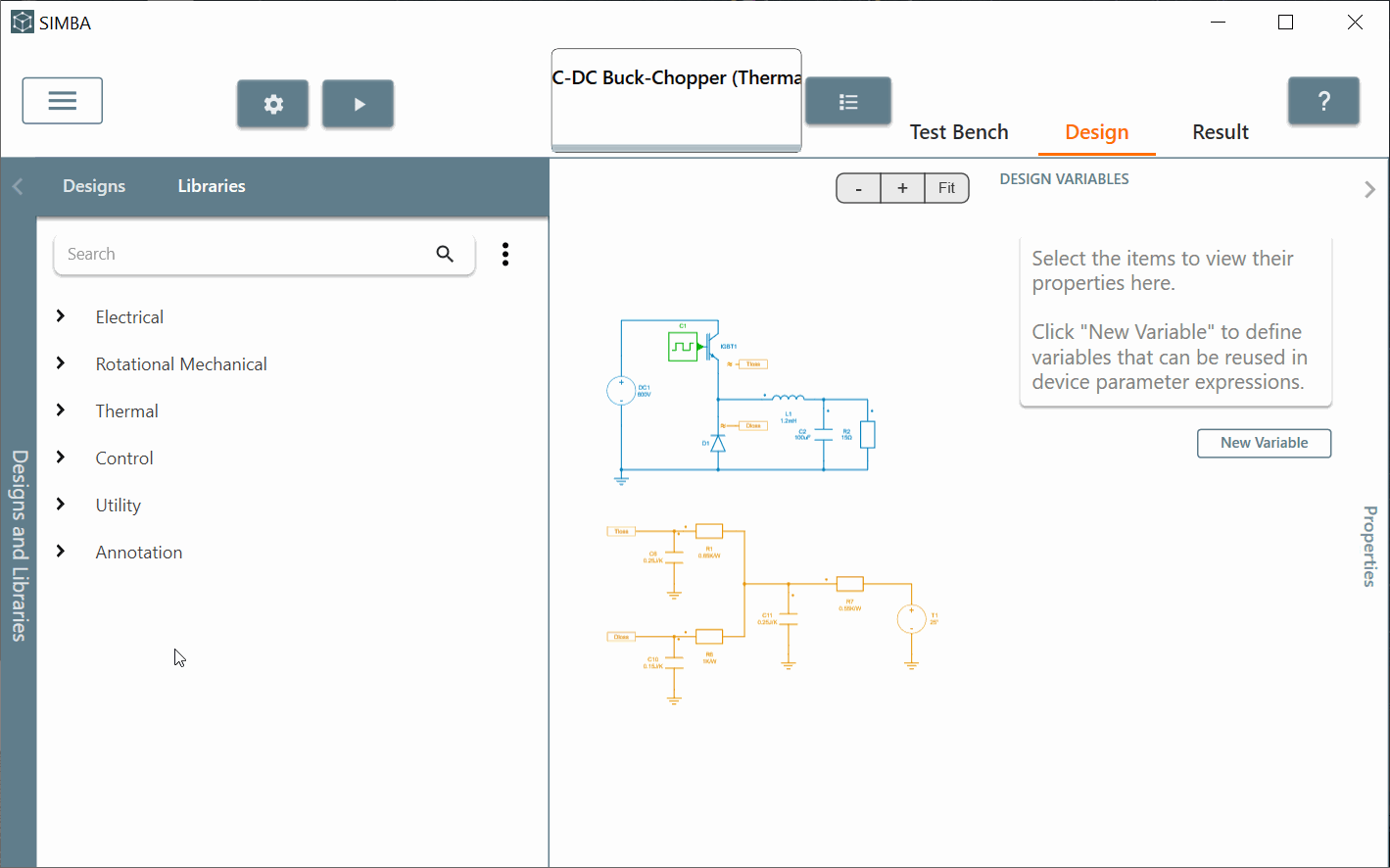Step 2: Secondly, let's check the Buck-Chopper power converter and more precisely the IGBT first.

Step 2.a: If you click on it you'll observe the Ron and Forward voltage which are equal to 0 for ideal behavior. But the main information to check are "Thermal Data" and "Average loss calculation frequency". Those 2 sections allow to select the thermal datas which need to be used during the simulation and the frequency value under which the average losses are calculated. Of course some scopes are availables for losses, energy and junction temperature calculation.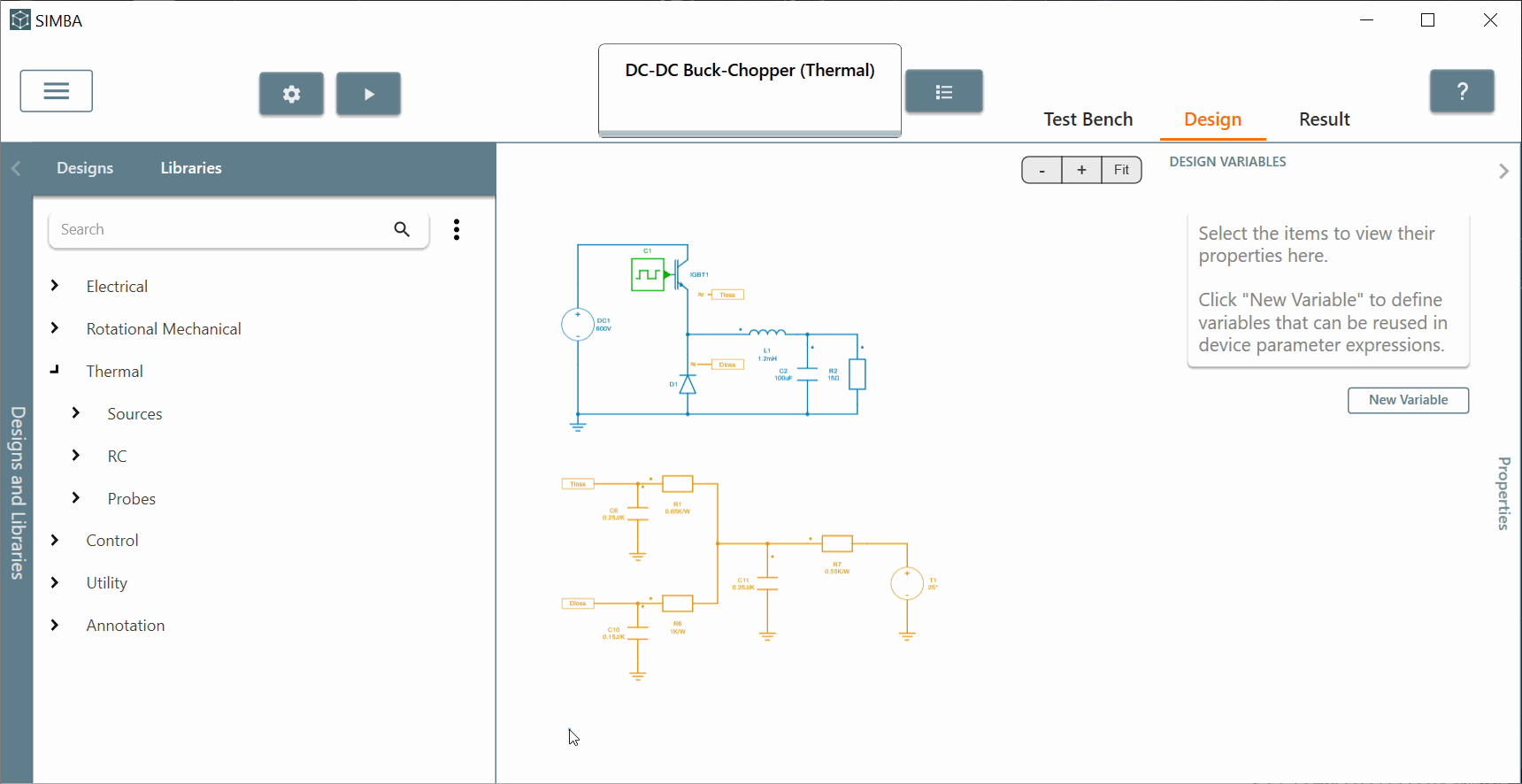Step 2.b: In order to set up thermal datas for losses and temperature purpose, you'll need to click on "Test Bench" tab and "Thermal Data" section. From there you can:

• import Thermal library file in .xml format that is currently supported by many semiconductors manufacturers. You can find some IGBT examples below: https://www.hitachienergy.com/products-and-solutions/semiconductors/stakpak
• create your own thermal component by clicking on "new" button. You'll then be able to fill in all the datas needed.
• modify and edit the actual thermal datas provided.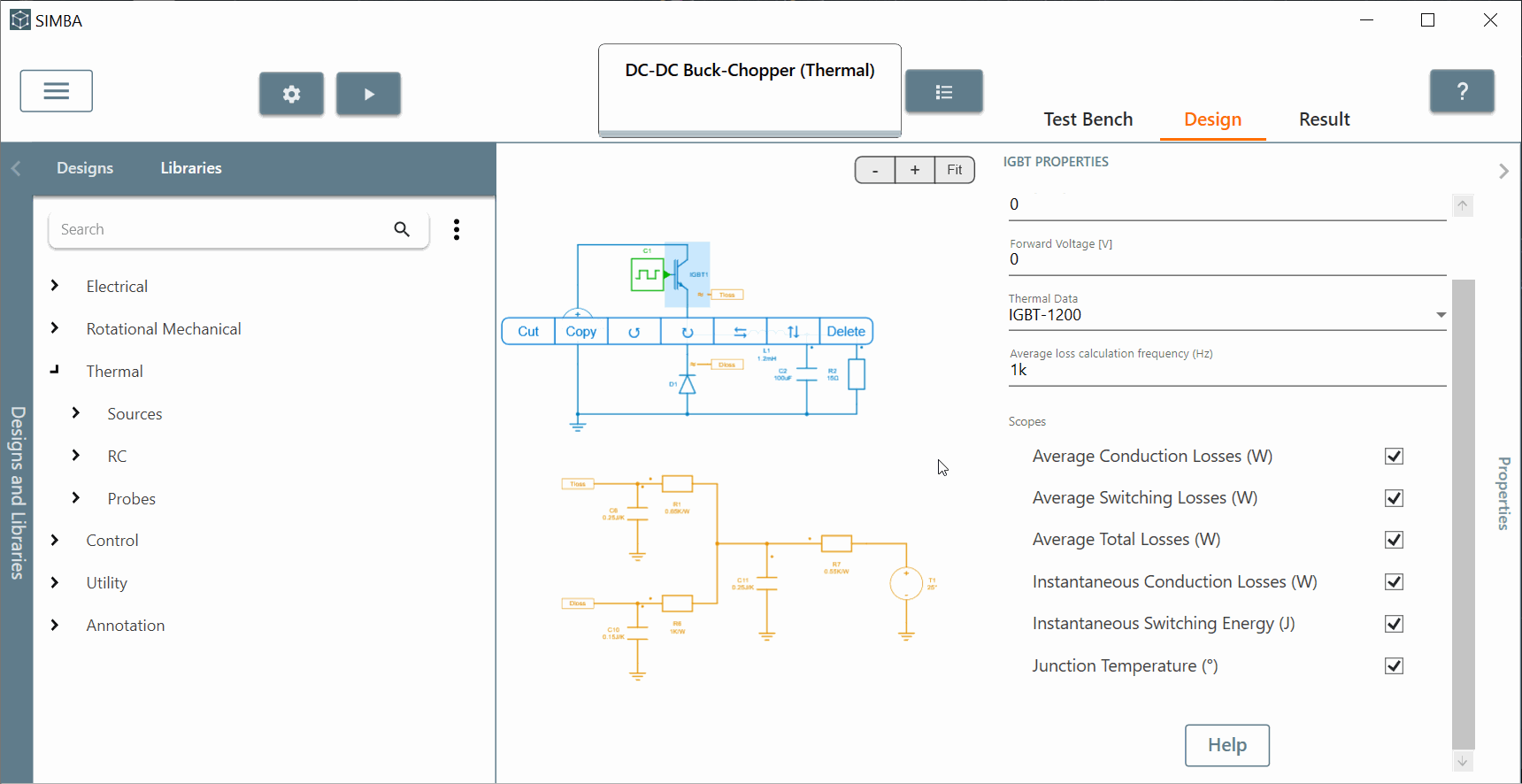Step 2.c: For any new devices added to the list, you can observe some section to fill in:

• Thermal impedance: you can decide between cauer of foster network for the junction to case thermal interface. You will need to specify the number of thermal resistors and capacitors representating the number of layers.

• custom Tables and variables : you can create any new variables and even tables in order to use them later in formulas and get a more realistic losses behavior. The values of the custom variables are defined by the user in the device properties.

• conduction loss: you'll need to fill in the data necessary for calculating conduction losses. You can decide between, lookup table, formulas and even both. You can add manually each datas (temperature, number of points, the datas of the lookup tables) or import them from a.csv file. The graph will be created and modified accordingly.

• Turn-On and Turn Off loss: you'll need to fill in the data necessary for calculating turn on and off losses. You can decide between, lookup table, formulas and even both. You can add manually each datas ( voltage and temperature, number of points, the datas of the lookup tables) or import them from a.csv file. The graph will be created and modified accordingly.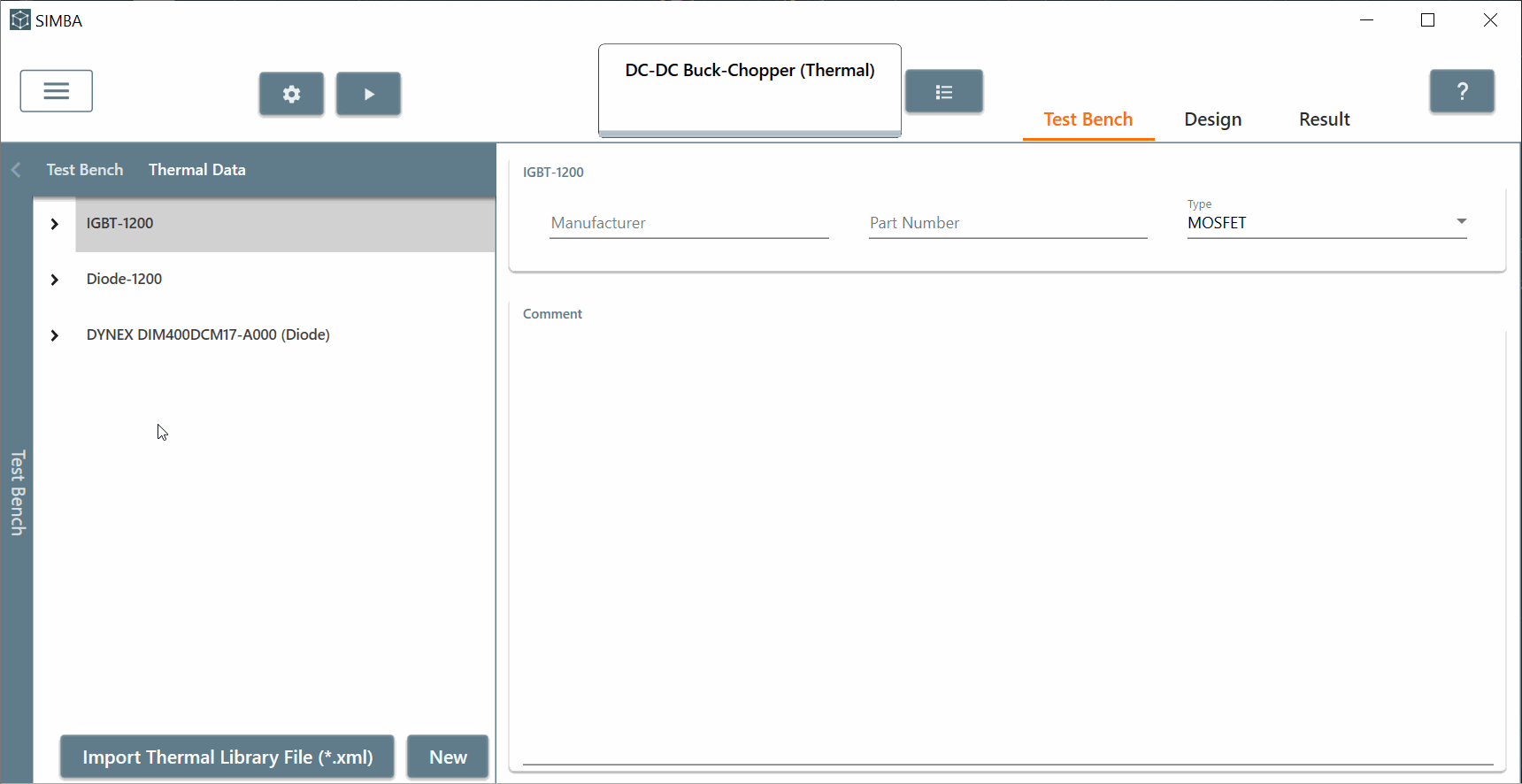Once a thermal data description has been created, it can be assigned to a power semiconductor switch (IGBT, MOSFET or diode) from schematic.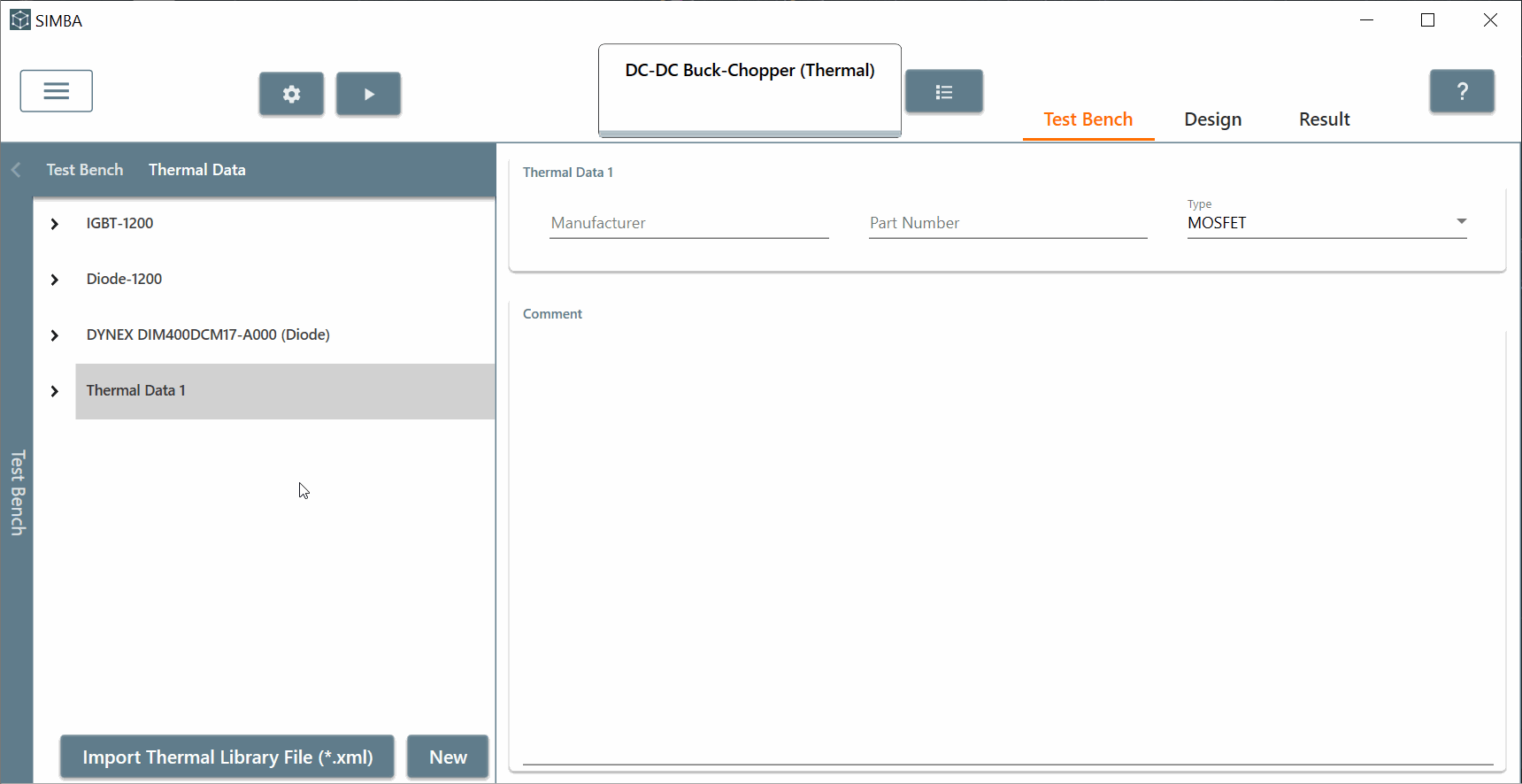Step 3: Thirdly, let's go back to the overall circuit. As you can see, when selecting thermal datas for both IGBT and Diode in the "properties" window, an additionnal pin is added. Conduction and switching losses of a power semiconductor have to be collected and connected to a thermal circuit to determine the junction temperature of the power semiconductor.
So the goal of this extra pin allows to connect the semiconductors to thermal circuit and provide the junction temperature as well. Then an orange thermal circuit is designed allowing the user to implement Thermal impedance and Heatsink Network until ambiant temperature. Of course some RC circuits are used for this purpose and measurements such as "Heat flow" and "Temperature" could be checked.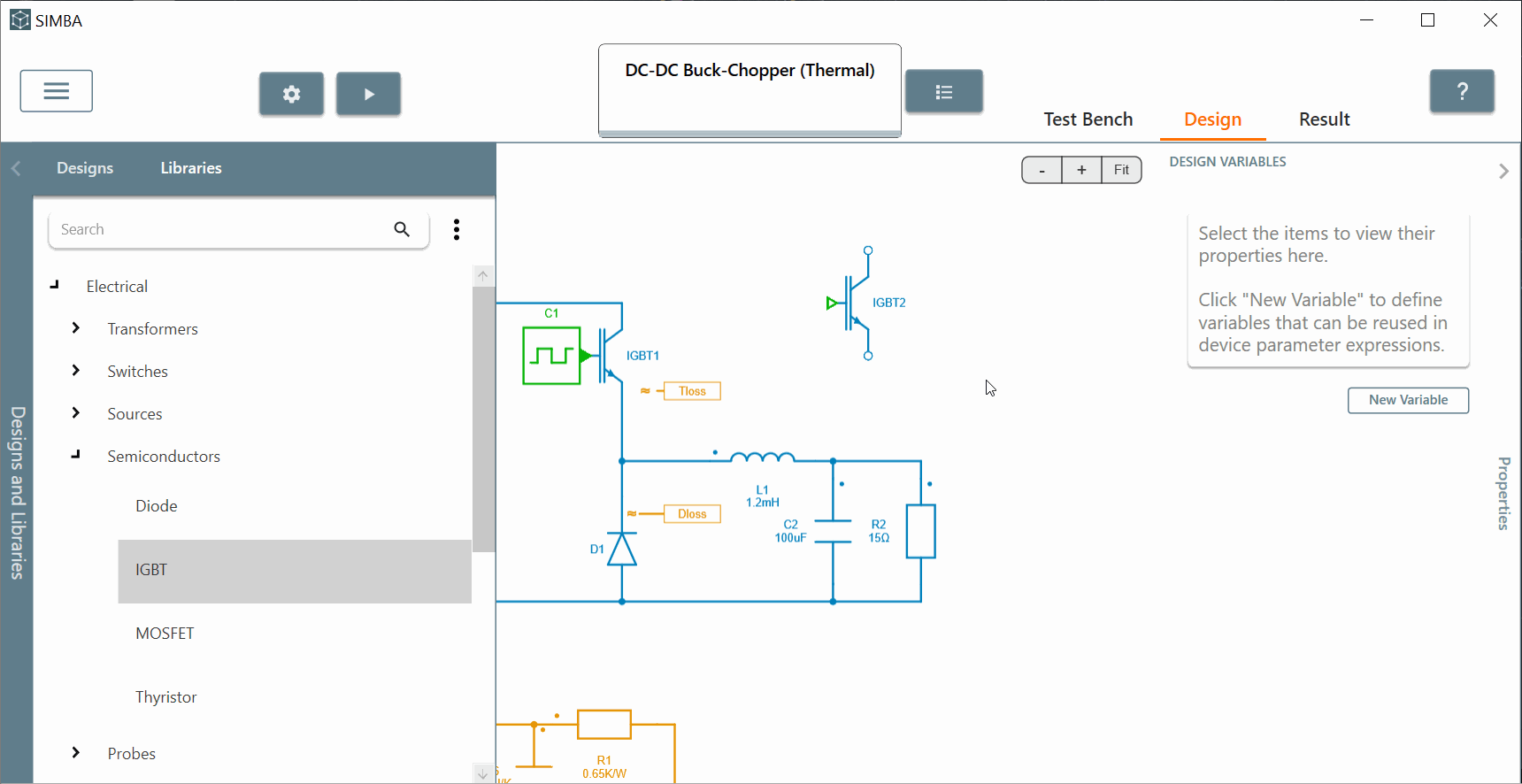Step 4: Finally, let's click on "IGBT" component, select all the scopes and run the circuit with original solver settings. We can for example display the "Average total losses" and the junction temperature . We can conclude that temperatures of IGBT increases a lot during the simulation and average total losses are quite stable during steady state. All those results are computed because of thermal datas entered by the user. Moreover Delaunay's triangulation and a barycentric interpolation are used to calculate the losses from those provided datas.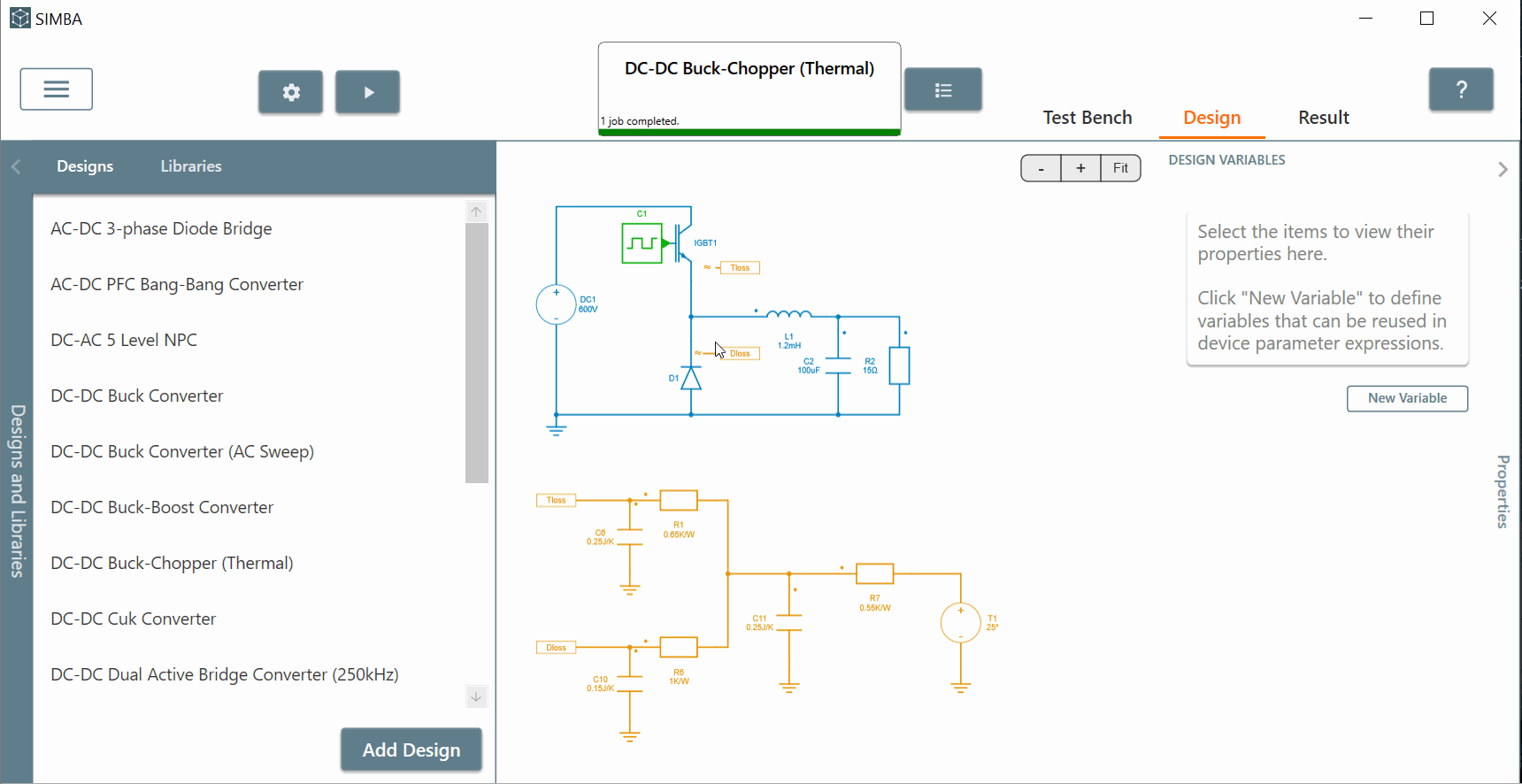More information about "Thermal Losses" calculation could be found on SIMBA website at "https://simba.io/doc/" --> "Thermal analysis" section.

This conclude this tutorial video about "Thermal modeling of power switches" feature of SIMBA.